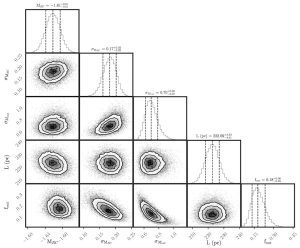# Gaia and the Red Clump

Authors: K. Hawkins, B. Leistedt, J. Bovy, D. W. Hogg

First Author’s Institution: Department of Astronomy, Columbia University, New York

Status: Published in MNRAS, open access

Red clump (RC) stars are common stars, once similar to the sun, that have evolved into red giants now supported by helium fusion in their cores. Independent of their exact age or composition, all RC stars end up having about the same absolute luminosity. This is why they tend to “clump” in a particular spot in a color-magnitude or Hertzsprung–Russell diagram (Figure 1), and what makes them standard candles: The apparent brightness of RC stars is directly related to their distance.Figure 1: A color-magnitude diagram of stars with well-measured parallax distances that are found in both the TGAS and 2MASS catalogs. – Reproduction of Figure 1 from the paper

As standard candles, RC stars have been used to measure distances to stellar associations in the Milky Way, as well as nearby galaxies. However, such measurements require a prior calibration, for which the reference brightness of known RC stars with known distances needs to be determined in the wavelength band used, and corrected for interstellar extinction. The authors of today’s paper carry out this calibration for a number of widely used bands, carefully taking into account the involved measurement uncertainties, and quantify just how useful RC stars are as standard candles.

The authors start by selecting a sample of known RC stars from multiple available catalogs, which include RC stars at various distances throughout the Milky Way (most of which have been identified using high-resolution infrared spectroscopy). They then retrieve the (uncalibrated) apparent magnitudes of these stars measured in a total of 8 optical to mid-infrared bands from the 2MASS, WISE and Gaia catalogs, and their trigonometric parallaxes from the Tycho-Gaia Astrometric Solution. Stellar parallax allows a purely geometric distance measurement, which is very reliable, but only feasible for relatively nearby stars. In total, 972 RC stars satisfy all the quality criteria imposed by the authors, for instance concerning the parallax precision.Figure 2: A graph that represents the authors’ model. The shaded circles indicate observed variables, the open circles indicate model parameters to be inferred, and the filled circles indicate parameters that are fixed. The absolute magnitude ($M_i$) of an observed RC star should be distributed around the intrinsic magnitude of the red clump ($M_\text{RC}$) with small scatter ($\sigma_\text{RC}$). The star could also be part of the fraction ($f_\text{out}$) of outliers, showing a larger scatter ($\sigma_\text{out}$). In any case, the interstellar extinction towards the star ($A_i$) and its distance ($r_i$) are needed to determine its observed apparent magnitude ($m_i$) and parallax ($w_i$), accounting for prior information about the distance ($L$). – Figure 2 from the paper

Next, the authors infer the absolute magnitude of the RC in each wavelength band, which is the one brightness that all RC stars should have, if they were at the same standard distance and perfect standard candles. Perhaps just as important is to estimate how precisely this absolute magnitude can be determined. By means of using a hierarchical probabilistic model (Figure 2), the authors are able to consistently account for the natural luminosity variation among RC stars, as well as measurement uncertainties related to the observed magnitudes, parallaxes, and the extinction correction, which is based on a 3D Galactic dust map. They can also account for the possibility of having falsely included some red giants in their sample that are not actually RC stars, by modeling these as a distinct population of outliers.

The authors’ final estimate for the absolute magnitude of the RC (Figure 3) is in fair agreement with previous studies across all bands, and the magnitude uncertainty implies a typical distance precision between 5% and 10%. Based on these findings, the authors conclude that RC stars are indeed reliable and precise standard candles. They also note that a specific advantage of using their hierarchical model is that the initial constraints on the parallaxes of distant stars are improved (i.e.$1/r_i$ is generally better constrained than$w_i$). This “error shrinkage” is a consequence of simultaneously inferring the properties of all stars together with the properties of their population.Figure 3: The inferred probability distributions of the model parameters (see Fig. 2), in the case of the 2MASS Ks band – Figure 3 from the paper

Next year’s second Gaia data release is expected to provide a significantly larger sample of RC reference stars. In particular, this will allow a closer study of the impact of such effects as stellar age and metallicity, helium abundance, or binarity on the RC distance calibration, and thus make RC stars even better standard candles.